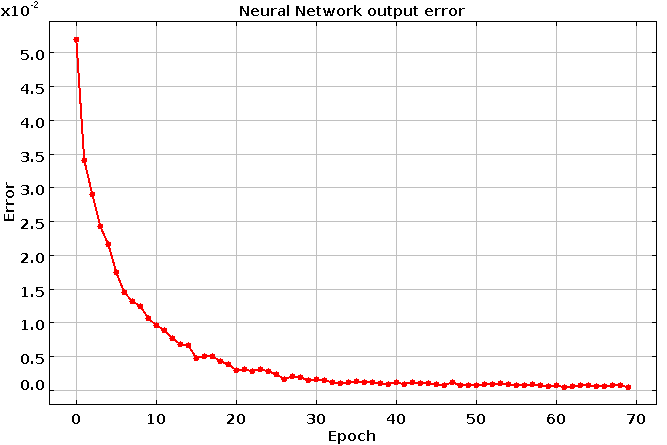# DMelt:AI/6 Classification problem

Limitted access. First login to DataMelt if you are a full DataMelt member. Then login to HandWiki as a user.

# Classification problem

Data classification is the central data-mining technique used for sorting data, understanding of data and for performing outcome predictions Data classification problem can be solved using statistical algorithms discussed in Section Statistical classification, as well as using Machine_learning which will be discussed in this section.

# Classification using neural networks

In this small tutorial we will use a library "Smile" (https://github.com/haifengl/smile) that includes many methods for supervising and non-supervising data classification methods. We will make a small Python-like code using Jython top build a complex Multilayer Perceptron Neural Network for data classification. It will have large number of inputs, several outputs, and can be easily extended for cases with many hidden layers. We will write a few lines of Jython code (most of our codding will deal with how to prepare an interface for reading data, rather than with Neural Network programming).

First of all, let us copy data samples. One sample will be for training (https://datamelt.org/examples/data/usps/zip.train), another file is for testing ((https://datamelt.org/examples/data/usps/zip.test). Copy these files to your local directory.

We will need to import the necessary classes to be used in this example:

from smile.data import AttributeDataset,NominalAttribute
from smile.data.parser import DelimitedTextParser,IOUtils
from smile.classification import NeuralNetwork
from smile.math import Math
from jarray import zeros,array
from jhplot import *
import java

We import the classes from several packages: "smile", "jhplot" and Java. Additional package "jarray" is used to work with Arrays using Jython. The NeuralNetwork class is the most important for our example. It creates Multilayer perceptron neural network that consists of several layers of nodes, interconnected through weighted acyclic arcs from each preceding layer to the following

We will call it as:

nn=[Nr_input, Nr_hidden, Nr_output] # define structure of NN
net=NeuralNetwork(NeuralNetwork.ErrorFunction.LEAST_MEAN_SQUARES, NeuralNetwork.ActivationFunction.LOGISTIC_SIGMOID,nn)
net.learn(x, y) # train neural net on input double array y, with the outcome given by x

Here we use the logistic sigmoid function and the error function "LEAST_MEAN_SQUARES".

Before start using this NN, we will read data from the files, and create input arrays x,y. We will write a small function "getJavaArrays" that returns two arrays, double[][] and int[] (using Java style). After NN was trained, we will use the test sample and call the method "predict(x)" to verify that our prediction are close to the expected values from the test sample.

The full code looks as this:

from smile.data import AttributeDataset,NominalAttribute
from smile.data.parser import DelimitedTextParser,IOUtils
from smile.classification import NeuralNetwork
from smile.math import Math
from jarray import zeros,array
from jhplot import *
import java

def getJavaArrays(dataset): # this function create x[][] and y[] array from datasets
rows=dataset.size()
lst = [0.0]*rows
twoDimArr = array([lst,[]], java.lang.Class.forName('[D'))
x = dataset.toArray(twoDimArr)
y = dataset.toArray(zeros(rows, "i"))
return x,y

parser =DelimitedTextParser(); parser.setDelimiter("[\t ]+")
parser.setResponseIndex(NominalAttribute("class"), 0)
train=parser.parse("Train",java.io.File("zip.train"))
x,y=getJavaArrays(train)   # get input and output for training NN

print "Rescale data range .. "
p = len(x)
mu = Math.colMeans(x);
sd = Math.colSds(x);
for i in range(len(x)):
for j in range(p):
x[i][j] = (x[i][j] - mu[j]) / sd[j];

nin=len(x);  nout=Math.max(y)+1
print "Training: Nr for input layer=",nin," Nr for output layer=",nout

nn=[nin,50,nout] # 50 nodes in a hidden layer. Need another layer? Add integer after 50
print "NN layout=",nn
net = NeuralNetwork(NeuralNetwork.ErrorFunction.LEAST_MEAN_SQUARES, NeuralNetwork.ActivationFunction.LOGISTIC_SIGMOID,nn)

c1 = SPlot() # plot error vs epoch
c1.visible();          c1.setGTitle("Neural Network output error")
c1.setAutoRange();     c1.setMarksStyle('various')
c1.setConnected(1, 0); c1.setNameX('Epoch'); c1.setNameY('Error')

for j in range(70):
net.learn(x, y)
error=0.0
for i in range(len(x)):
if (net.predict(x[i]) != y[i]): error +=1
error=error / len(x)
c1.update()
print "Epoch=",j," Error=",error
print "Testing using zip.test file.."
test=parser.parse("Test",java.io.File("zip.test"))
testx,testy=getJavaArrays(test)

for i in range(len(testx)): # rescale data
for j in range(p):
testx[i][j] = (testx[i][j] - mu[j]) / sd[j];

error=0.0
for i in range(len(testx)):
if (net.predict(testx[i]) != testy[i]):  error +=1
print "Error rate =", 100.0 * error / len(testx),"%"

Note that we use 50 nodes in a single hidden layer. The number of input (256) and outputs (10) are given by the structure of the data (looks at the data file to see their structure).

Now you can run it using the DataMelt data program (https://datamelt.org/). Install DataMelt, open the above code in the editor and save these lines in a file with the extension ".py" (say, "test.py"). This is an important step, since tells DataMelt how to run this code. Then run this code: click the icon with a green running man in the top menu, or press [F8]. You will see an image which shows how the learning error shrinks as a function of epoch number. The last line of the code gives the error rate for your prediction.If you are interested in deep neural networks, increase the number of hidden layers using the input list "nn". For example, if you need a second hidden layer with 25 nodes, extend the list in the above example as:

nn=[nin,50,25,nout]

If you will see that the number of epochs will be drastically reduced to obtain a similar (or better) rate for successful predictions (but, the CPU time, will increase since more time will be needed to adjust the weights).

If you are interested in more examples using other methods to classify data, such as K-Nearest Neighbor, Linear Discriminant Analysis, Fisher's Linear Discriminant, Quadratic Discriminant analysis, Regularized Discriminant Analysis, Logistic Regression, Maximum Entropy Classifier etc, look this link: https://datamelt.org/code/index.php?art=Data_mining/Classification which shows how to use such methods using the Python interface.